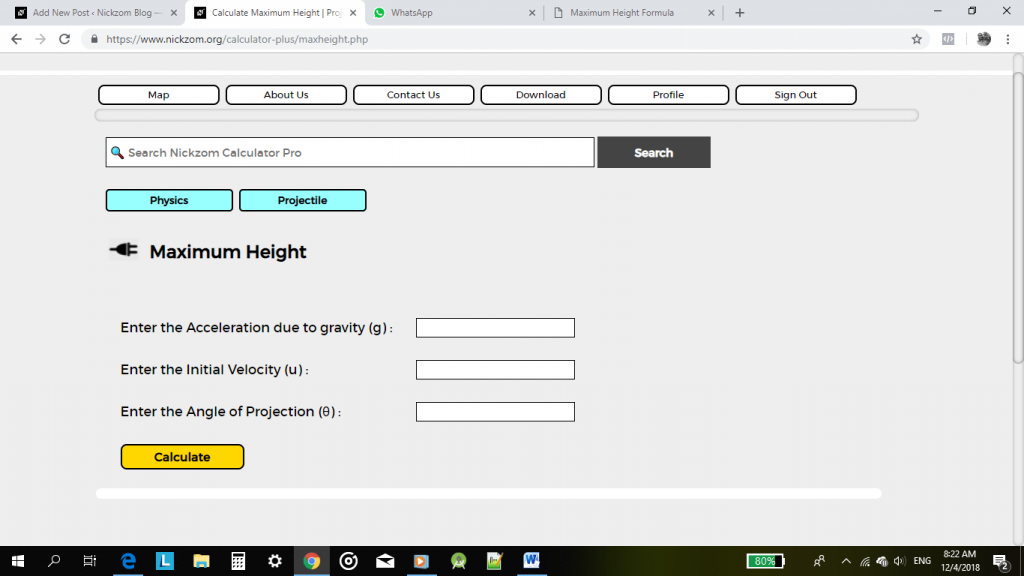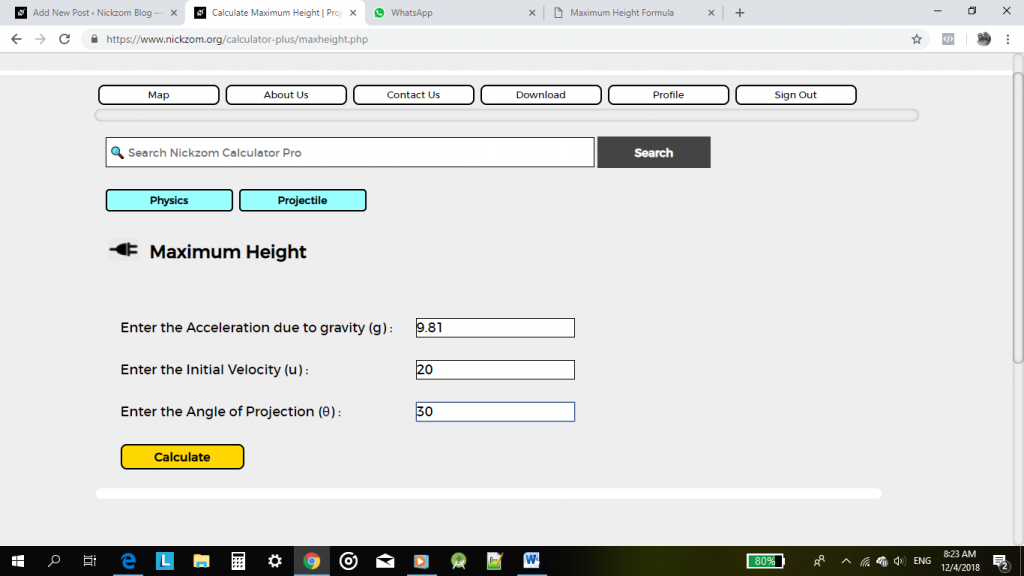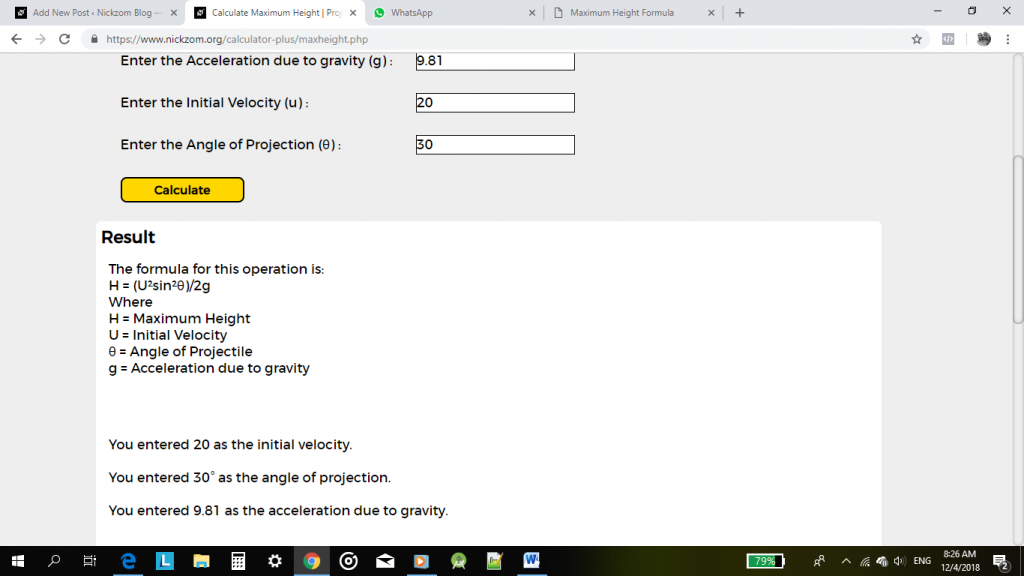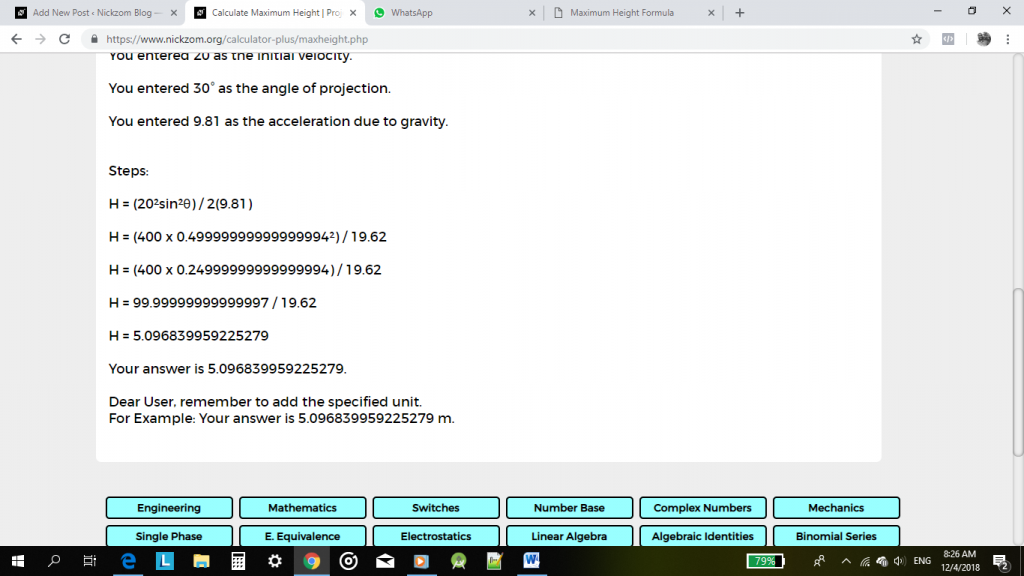# Nickzom Calculator Calculates Maximum Height of a Projectile

According to SoftSchools.com,

A projectile is an object that is given an initial velocity, and is acted on by gravity. The maximum height of the object is the highest vertical position along its trajectory. The maximum height of the projectile depends on the initial velocity v0, the launch angle θ, and the acceleration due to gravity. The unit of maximum height is meters (m).

Nickzom Calculator calculates the maximum height of a projectile given the accurate values for the required parameters. The required parameters to get the answer to maximum height are:

• Acceleration due to Gravity
• Initial Velocity
• Angle of Projection

Let’s get started, first and foremost, proceed to the home page of the app, select Physics and click on Open Section.Now, click on ProjectileClick on Maximum HeightThe interface to enter the values for the parameters.Let’s say you want to calculate the maximum height of a projectile with an acceleration due to gravity of 9.81 m/s2, initial velocity is 20 m/s and angle of projection is 30°.Now, click on CalculateThe formula, workings and answer to the projectile is now displayed for you.

You can access Nickzom Calculator (The Calculator Encyclopedia) via any of these channels:

## One thought on “Nickzom Calculator Calculates Maximum Height of a Projectile”

1.تقييم التطبيقات says:

I’ve been surfing on-line greater than 3 hours nowadays, yet I never discovered any attention-grabbing article like yours.
It’s lovely price sufficient for me. In my view, if all web owners and bloggers
made good content as you probably did, the net will likely be much
more useful than ever before.